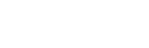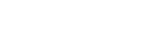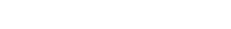## MATHEMATICS PAPER 1 - KCSE 2019 JOINT PRE MOCK EXAMINATION NAMBALE

1. Evaluate without using a calculator (2 Marks)1. In Blessed Church choir, the ratio of males to females is 2:3. On one Sunday service, ten male members were absent and six new female members joined the choir as guests for the day. If on this day the ratio of males to females was 1:3, how many regular members does the choir have? (3 Marks)
1. A Kenyan bank buys and sells foreign currency as shown below.
Kenya shillings            Kenya shillings
1 Euro                                84.15                           84.26
1 US Dollar                         80.12                           80.43
A tourist travelling from Britain arrives in Kenya with 5000 Euros. He converts all the Euros to Kenya shillings at the bank. While in Kenya he spends a total of KSh. 289,850 and then converts the remaining Kenya shillings to US dollars at the bank. Calculate (to nearest dollar) the amount he receives? (3 Marks)
1. Simplify the expression. (3 Marks)1. Complete the figure below so as to make the net of a cuboid. Hence determine the surface area of the cuboid. (4 Marks)1. The sum of the interior angles of a regular polygon is 10800. Calculate
1. The number of sides of the polygon (2 Marks)
2. The sizes of the exterior and interior angles of the polygon.             (2 Marks)
1. If 32x-4(3x)+3=0 . Find the possible values of x (3 Marks)
1. Three similar pieces of timber of length 240cm, 320cm and 380cm are cut into equal pieces. Find the largest possible area of a square which can be made from any of the three pieces. (3 Marks)
1. The sum of digits formed in a two digit number is 16. When the number is subtracted from the number formed by reversing the digits, the difference is 18. Find the number (3 Marks)
1. Solve for x given that                                                 (3 Marks)1. Three pens and four exercise books cost Sh. 87. Two pens and five exercise books cost Sh. 93. Find the cost of one pen and one exercise book. (4 Marks)
1. A farmer has enough feed to last 45 cows for 30 days. If he buys 5 more cows, how long will the feed last?   ( 2 Marks)
1. Find the equation of the line perpendicular to 3x – 7y – 20 = 0, and passes through the point (5,2) (3 Marks)
1. Wanza sold a bag of potatoes for Sh. 420 and made a profit. If she sold it at Sh. 320, she could have made a loss. Given that the profit is thrice the loss, how much did she pay for the bag of potatoes? (3 Marks)
1. In the figure below PQRS is a trapezium with QR parallel to PS. QR = 6cm, RS = 4cm, QS = 9cm and PS = 10cm.Calculate
1. The size of angle SQR                                                                                                             (2 Marks)
2. The area of triangle PQS                                                                                                         (2 Marks)
2. Given that Cos (x – 20)0 = Sin (2x + 32)0 and x is an acute angle, Find tan (x – 4)0             (3 Marks)

SECTION II (50 MARKS)
Answer Only Five Questions In This Section

3. An expedition has 5 sections AB, BC, CD, DE and EA. B is 200m on a bearing of 0500 from A. C is 500m from B. The bearing of B from C is 3000. D is 400m on a bearing 2300 from C. E is 250m on a bearing 0250 from D.
1. Sketch the route                                                                                                                       (1 Mark)
2. Use the scale of 1cm to 50m to draw the accurate diagram representing the route.               (5 Marks)
3. Use your diagram to determine
1. Distance in metres of A from E                                                                                         (2 Marks)
2. Bearing of E from A
4. A business lady bought 100 quails and 80 rabbits for Sh. 25,600. If she had bought twice as many rabbits and half as many quails she would have paid Sh. 7,400 less. She sold each quail at a profit of 10% and each rabbit at a profit of 20%.
1. Form two equations to show how much she bought the quails and the rabbits                      (2 Marks)
2. Find the cost of each                                                                                                              (3 Marks)
3. Calculate the profit she made from the sale of the 100 quails and 80 rabbits                         (3 Marks)
4. What percentage profit did she make from the sale of the 100 quails and 80 rabbits                        (2 Marks)
5. The table below shows the length of 40 seedlings.
Determine
1.
1. The modal class         (1 Mark)
2. The median class        (2 Marks)
2.
1. The mean of the seedlings                                                                                                  (4 Marks)
2. The median of the seedlings                                                                                        (3 Marks)
6. Find1. The surface area of the frustrum       (5 Marks)
2. The volume of frustrum shown.     (5 Marks)
1. Triangle ABC vertices A (-2, 6), B (2, 3) and C (-2, 3) is reflected in the line x = -3 to give the image A1B1C1. A1B1C1    is translated by the vector(10, 9) to give image A2B2C2. A3B3C3 with coordinates A3 (6,-6) B3 (2,-3) and C3 (6,-3) is the image of A2B2C2 after transformation. Plot all the triangles in the grid provided and determine
1. The transformation that maps A2B2C2onto A3B3C3                                                                (2 Marks)
2. The simple transformation that maps ABC onto A3B3C3                                                       (2 Marks)
1. In the figure below AOC is a diameter of the circle centre O; AB = BC and ÐACD = 350. EBF is a tangent to the circle at B. G is a point on the minor arc CD.Giving reason
1. Calculate the size of
2. The obtuse ÐBOD                                                                                                            (2 Marks)
3. ÐBGD                                                                                                                              (2 Marks)
2. Show that ÐABE = ÐCBF                                                                                                    (3 Marks)
1. The diagram below shows the speed-time graph for a bus travelling between two stations.The bus begins from rest and accelerates uniformly for 30 seconds. It then travels at a constant speed for 60 seconds and finally decelerates uniformly for 40 seconds.
Given that the distance between the two stations in 2090m. Calculate
1. The maximum speed, in km/h the bus attained                                                                       (3 Marks)
2. The acceleration                                                                                                                      (2 Marks)
3. The distance travelled during the last 20 seconds                                                                   (2 Marks)
4. The time the bus takes to travel the first half of the journey                                                  (3 Marks)
1. The members of a photograph club decided to buy a camera worth Shs. 4000 by each contributing the same amount of money. Fifteen member failed to pay their contribution due to various reasons. As a result each of the remaining members had to contribute Sh. 60 more.
1. Find the number of members in the club                                                                                (7 Marks)
2. What was the percentage increase in the contribution per month?                                         (3 Marks)## MARKING SCHEME#### Download MATHEMATICS PAPER 1 - KCSE 2019 JOINT PRE MOCK EXAMINATION NAMBALE.

• ✔ To read offline at any time.
• ✔ To Print at your convenience
• ✔ Share Easily with Friends / Students